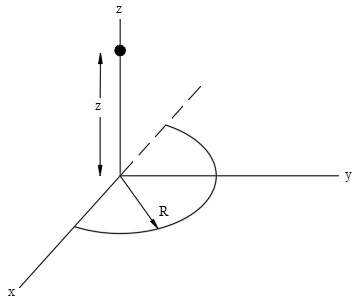# A thin semicircle of radius R carries a linear uniform charge density \lambda, as shown in the...

## Question:

A thin semicircle of radius R carries a linear uniform charge density {eq}\lambda {/eq}, as shown in the figure. The point P is at a distance z from the center of the semicircle along the z axis.

(a) What is the total charge of the semicircle?

(b) By integrating, calculate {eq}E_{z} {/eq}, the z-component of the electric field at point P.

(c) What is the potential at point P? Take the potential to be zero at infinity.

(d) Calculate {eq}E_{z} {/eq} at point P using the expression for the potential calculated in (c).

(e) How far from the origin must a charge -Q < 0 be placed so that the potential is zero at the origin?## Finding the electric field and electric potential due to a charge distribution

The electric field at a distance {eq}r {/eq} from a point charge {eq}q {/eq} is simply {eq}kq/r^2 {/eq}, where {eq}k {/eq} is Coulomb constant. If we are given a charge density instead of a point charge, then we can express an infinitesimal charge in terms of its charge density and an infinitesimal length. The total field is then the integration of the electric field due to all infinitesimal charge.

To find the potential, it is simply {eq}kq/r {/eq} if potential at infinity is set to be 0. Again, {eq}q {/eq} can be expressed in terms of charge density and length. When we obtain the potential, it is easy to find the electric field at this point, since the field is just the negative gradient of potential.

(a)

We are given the charge density {eq}\lambda {/eq} of a semicircle with radius {eq}R {/eq}. Suppose the total charge of this semicircle is {eq}Q {/eq}, then {eq}Q {/eq} can be expressed in terms of {eq}\lambda {/eq} and length of this semicircle {eq}l {/eq} or {eq}R {/eq}:

{eq}\begin{align*} Q&= \lambda l,\\ &= \lambda\pi R. \end{align*} {/eq}

(b)

We can find the electric field at point {eq}P {/eq} due to this semicircle by calculating the electric field due to all infinitesimal charge {eq}dQ {/eq}.

{eq}\begin{align*} E&=\int dE,\\ &=\int \frac{kdq}{d^2}, \end{align*} {/eq}

where {eq}d {/eq} is the distance from the infinitesimal charge {eq}dq {/eq} to the point {eq}P {/eq}. Inserting the result we obtained in part (a), this equation becomes

{eq}\begin{align*} E&= \frac{k \lambda}{d^2}\int dl,\\ &= \frac{k \lambda R}{d^2}\int_0^\pi d \theta,\\ &= \frac{k \lambda\pi R}{d^2}. \end{align*} {/eq}

Suppose {eq}\theta {/eq} is the angle between the line connecting {eq}P {/eq} and the infinitesimal length {eq}dl {/eq} and the {eq}z {/eq}-axis, then the {eq}z {/eq}-component of the electric field is just

{eq}\begin{align*} E_z&=E \cos\theta,\\ &=E \cdot \frac{z}{d},\\ &= \frac{k \lambda\pi Rz}{d^3},\\ &= \frac{k \lambda\pi Rz}{ \left(R^2+z^2\right)^{3/2}}. \end{align*} {/eq}

Note that since the semicircle is symmetric about the {eq}x {/eq}-axis, {eq}E_y {/eq} is canceled out, and only {eq}E_x {/eq} and {eq}E_z {/eq} are left.

(c)

If taking the potential to be zero at infinity, the potential at point {eq}P {/eq} due to this charge distribution is

{eq}\begin{align*} V&=\int \frac{kdq}{d},\\ &= \frac{k}{d}\int \lambda\,dl,\\ &= \frac{k \lambda R}{d}\int_0^\pi d \theta,\\ &= \frac{k \lambda\pi R}{d},\\ &= \frac{k \lambda\pi R}{ \sqrt{R^2+z^2}}. \end{align*} {/eq}

(d)

When we have obtained the electric potential at point {eq}P {/eq}, the {eq}z {/eq}-component of the electric field is the negative gradient of this potential along {eq}z {/eq}-axis.

{eq}\begin{align*} E_z&=- \frac{\partial V}{\partial z},\\ &=-\left(- \frac{1}{2}\right) \frac{k \lambda\pi R}{ \left(R^2+z^2\right)^{3/2}} \cdot 2z,\\ &= \frac{k \lambda\pi R z}{ \left(R^2+z^2\right)^{3/2}}, \end{align*} {/eq}

which is exactly the same as we have obtained in part (b).

(e)

The electric potential due to this semicircle at origin is when {eq}z=0 {/eq}, so

{eq}\begin{align*} V_O&= \frac{k \lambda\pi R}{R},\\ &=k \lambda\pi,\\ &= \frac{kQ}{R}. \end{align*} {/eq}

The potential at origin is like the potential due to a point charge {eq}Q {/eq} at a distance {eq}R {/eq} from origin. Hence, if we put a negative charge {eq}-Q {/eq} at a distance {eq}R {/eq} from origin, the electric potential is

{eq}\begin{align*} V&= -\frac{kQ}{R}, \end{align*} {/eq}

which can be canceled out the potential due to the semicircle.